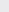Course Content

0/4

0/3

0/1

0/2

0/1

0/4

0/3

0/5

0/3

0/5

0/5

0/1

0/6

0/2

0/4

0/5

16.2 Arithmetic Progression 等差数列
1.
Given that the 11term of an arithmetic progression is 34, find the sum of the 3term, 9term and 21term.

1022.
Figure beside shows few circles where their radii increase by 1cm as follow on. Given that the total length of perimeter of the first five circles is 120cm, find the length of radius for the smallest circle.

10 cm
3.
The nterm of a arithmetic progression is represented as Tand the sum of first n terms is represented as S. Given that T+ T= 40 and S:S= 2 : 3.
T表示一等差数列的第n项而 S表示首n项的和。已知 T+ T= 40 和 S:S= 2 : 3.

(a) Calculate the value of the first term and the common difference.

(b) Find the value of S.

(a) 5, 2

(b) 1020

4.
Figure beside shows three right angle triangle which has a fixed base and height decreasing 2 units as follow on.

(a) Show that the area of the three triangles is an arithmetic progression.

(b) If the areas of the following triangles have the same characteristic as above, given the total area of first six triangles is 1620cm, find the area of the first triangle.

(c) Calculate the total area of all triangles that was formed.

(b) 300

(c) 3900cm5.
Find the sum of all integers divisible by 4 and 7, lying between 400 and 700.

Given that the first term, a and the common difference, d of an A.P. have the relation such that d – a = 1. If S= 610, S– S= 30, where S= T+ T+ T+ … +Tand S= T+ T+ T+ … +T= T+ T+ … + T, find the values of a, d and n.
Given that in an A.P., the pterm is 2000, the 2000term is p. Find the (2000 + p)term.